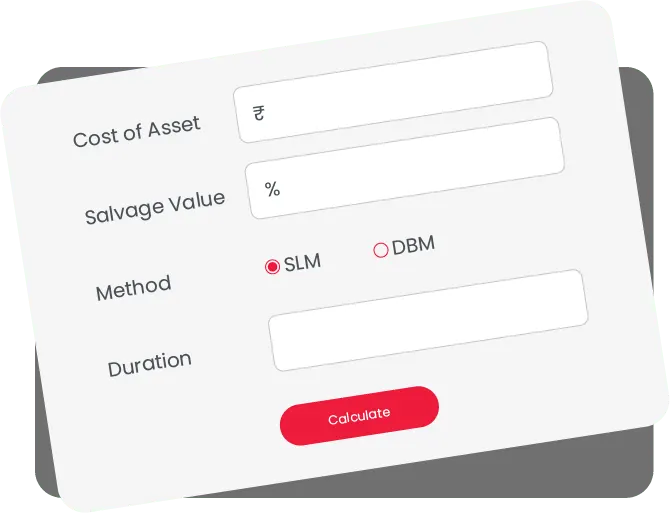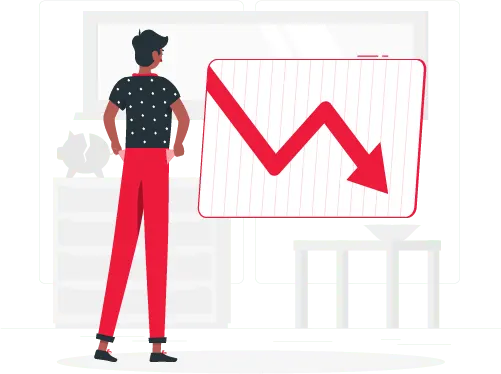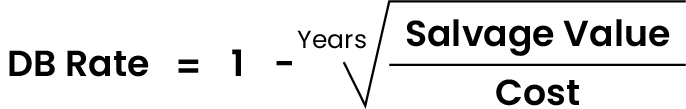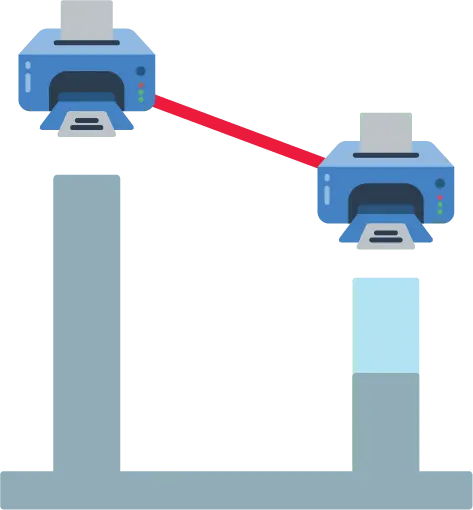# Online Depreciation Calculator India

Please provide a valid Net Price
Asset Cost cannot exceed 1,00,00,000
%
Salvage Value cannot be more than 100%
Please provide a valid salvage value
Duration cannot be more than 20 years
 Year Opening Value Depreciation Closing Value

## Depreciation calculator

A small business can quickly write off its expenses whenever they occur. However, it isn’t the case for fixed asset items like vehicles and buildings. It is when you come across the usage of an accounting term called depreciation. It is used to spread the value of an asset over the years. But, what does depreciation mean? You can understand the depreciation of an asset quantitatively by using a depreciation calculator.Can a business accounting software help in calculating the correct value of depreciation? You will find out the answer to all your queries regarding depreciation in this article. Further, you can use the Vyapar depreciation calculator online to confirm the depreciation value of your asset.### What is Depreciation in Accounting?

In terms of accounting, depreciation is a systematic reduction of the cost of any fixed asset till it becomes useless, or in other words, its value becomes negligible. Some examples of fixed assets that depreciate are furniture, machinery, equipment, etc. The land is an exceptional fixed asset that appreciates over time. Further, businesses might want to adjust the depreciation rate in a particular manner to write off more value in the initial years. You can list up your assets in our business accounting software, and the Vyapar depreciation calculator will help evaluate your asset’s correct depreciation value.

Using depreciation expenses while filing taxes help you gain better control over your business finances. Different assets are sorted into different classes to understand their useful life better. A business can decide the asset’s useful life based on the life expectancy for that asset. A depreciation calculator can help deduce the effective depreciated value of an asset over its lifetime. For example, one might suggest computer equipment depreciate over five years, but if your business requires renewal every three years, you can appreciate it over a shorter time.

An example to understand depreciation:
A trucking company purchases a truck with a cost of INR 2,50,000. The expected usage of trucks is five years so that the business can depreciate the asset under a depreciation expense of INR 50,000 every year for five years. Some companies might want to adjust the depreciation rate to write off more value in the initial years to get the maximum rebate earlier.

## How to calculate depreciation using a Depreciation Calculator?

You can quickly get depreciation values using the Vyapar depreciation calculator tool. To calculate depreciation, some key information that is required is as listed below:1. Useful life: The time for which an asset is considered productive for a business is termed as its useful life. In most cases, once the useful life of an asset is over, the asset is no longer cost-effective or efficient for the business.
2. Salvage value: Once the asset gets depreciated, a business might want to sell it over at a reduced rate. The amount that can get fetched on selling the asset is its salvage value.
3. Cost of asset: The complete cost of purchasing, installing, and shipping an asset is termed as its cost.

Using depreciation in yearly accounting records ensures that they report the correct profit values in their income statement and balance sheet. It is essential to fill in the depreciation details correctly, so let your company’s accountant handle calculations related to depreciation. You can use Vyapar business accounting software to manage the depreciation calculations automatically.

## Methods of Depreciation Calculation

There are many ways to calculate depreciation for your financial sheets. However, the IRS requires a business to use only one method of depreciation while filing tax returns. So, some companies choose to have a single depreciation method for both financial books and tax returns. However, some businesses use one method for books and the other for tax purposes.
Here are the four different methods of depreciation that are widely popular:

Straight-line method of depreciation

The simplest way to depreciate any fixed asset is a straight-line method of depreciation. It is used widely in the industry as it simply distributes the value of an asset over its usable life. It is the best-suited method for small businesses with a smaller team. Mostly the companies with no tax advisor or accountant in the team.
Formula:
Depreciation = (asset cost – salvage value) / useful life
Example:
Your driving business buys a taxi for INR 5,00,000, and the salvage value of the taxi is 50,000 after ten years of use. We can use the data in the equation to get depreciation.

(5,00,000-50,000) / 10 = 45,000
So, you have to write off 45,000 from the taxi’s value every year for ten years.

Declining Balance Method of Depreciation:

The double-declining balance method is a slightly complicated way of depreciating an asset. Using this method, you can write off more of the asset’s value in the initial years and less later on. It is best when a business wants to recover more value of their asset upfront.
Formula:
For first year –
Yearly Depreciation Value = (2 x straight-line depreciation rate) x (book value at the beginning of the year)
For next year and so on-
Yearly Depreciation Value = (2 x straight-line depreciation rate) x (book value at the beginning of the year (after deduction of the last year depreciation))
Example:
Using the taxi example above, we can determine that the asset gets depreciated over ten years.
So, its depreciation rate is 10%. Using it in the equation.
Year 1 depreciation = (2 x 0.10) x 5,00,000 = 1,00,000
Now, after writing off 1,00,000 for the first year, the book value of the taxi is 4,00,000.

Year 2 depreciation = (2 x 0.10) x 4,00,000 = 80,000
Now, after writing off 80,000 for the first year, the book value of the taxi is 3,20,000.

Similarly, you can calculate the depreciation value for every year after that till Year 10.

Sum-of-the-Years-Digits (SYD) Method of Depreciation

The sum-of-the-year’s-digits (SYD) depreciation is another popular depreciation method that allows businesses to hive off more cost of any asset in the early years of asset purchase. It is great for those businesses who want to have a slightly more even distribution of an asset’s value compared to the double-declining balance method.
Formula:
Yearly Depreciation Value = (remaining lifespan / SYD) x (asset cost – salvage value);
Where SYD is the addition of digits of each year of an assets useful life. Example:
Sticking with our taxi example, we can calculate SYD.
SYD value = 1 + 2 + 3 + 4 + 5 + 6 + 7 + 8 + 9 + 10 = 55.
Using SYD in the equation.

Year 1 depreciation = (10 / 55) x (5,00,000 - 50,000) = 81818.18
Write off 81818.18 for the first year.

Year 2 depreciation = (9 / 55) x (5,00,000 - 50,000) = 73636.36
Write off 73636.36 for the second year.
Similarly, you can write off depreciation every year accordingly.

Units of Production Method of Depreciation

The best way to depreciate an asset according to the amount of work it does is the unit of production method depreciation. By “units of production,” you can either take the number of products it creates or the number of active service hours. It is best suited for businesses whose equipment’s depreciation is heavily dependent on the amount of time it works. Using this method requires tracking the use of equipment, so it is mostly suited for high-value machinery.
Formula:
Current year depreciation value = (asset cost – salvage value) / units produced in useful life
Example:
Let’s stick to our taxi example and to calculate how much work it does.
We can use the distancetravelled by it in kilometers. Assume that a taxi gets depreciated after 1,00,000 km.

For depreciation cost, we divide the taxi’s book value with units of production
4,50,000 / 1,00,000 = 4.5
So, the taxi has a depreciation of INR 4.5 for every km

Let’s assume in the first year; the taxi travelled 10,000 km.
10,000 x 4.5 = 4,500
We can write off 4,500 depreciation for the first year.
Similarly, the depreciation of each year is calculated till the production limit reach (1,00,000 km travel in this example)## Partial Year Depreciation

Assets are purchased and retired any time throughout the year. So, it gets difficult for businesses to determine how much depreciation expense they should report. So, to get a clear picture, a partial year’s depreciation is calculated. Some of the conventions followed to determine partial year’s expense are:

1. Total Workdays of an equipment
2. Actual months used (full month or half month variations)
3. Quarterly or half-yearly charge distribution
4. Full-year charge in year of acquisition and none in year of disposal

## Depreciation calculator is primarily used for

You can use our depreciation calculator for any one of the listed reasons. It will help you find the most suited real estate.

Apartment depreciation calculator India

An apartment depreciation calculator is used to understand the value of an apartment at a specific point in time after the construction is completed. The value of a built structure decreases due to a reduction in the usable life span of the building. However, as apartments share common space among multiple units, land value appreciation is equally distributed among all flats within reach, making it smaller than a house. You can factor in your share of the appreciated land value and depreciated structure value using an apartment depreciation calculator to get the final apartment value.

Furniture depreciation calculator India

Calculate the depreciation value of your furniture by using the furniture depreciation calculator by Vyapar. Using our app, you can spread the cost of acquiring furniture for your business over its lifetime in your balance sheet. Using depreciation, you can make your balance sheet cleaner and get tax rebates for your purchase over the lifetime of the furniture. By utilising a furniture depreciation calculator, you can speed up the calculation process. Further, if you wish to sell your furniture in future, you can quote the depreciated value as a price for the sale.

Property depreciation calculator India

Many businesses and individuals purchase property as an investment as the underlying land value appreciates over time. Using a property depreciation calculation, you can combine the land appreciation price with the property cost depreciation to find the final property price at any point in time. If you have purchased a property purely for the land, it will continue to appreciate over time. However, if there is a structure present on it, the value of that structure will get depreciated over its lifetime. Using a property depreciation calculator, you can calculate the fair value of your property.

House depreciation calculator India

House depreciation is a standard cost deduction process that is used effectively when making a purchase of any house. If you rent out your home, you can deduct the rental income you earn from the house depreciation cost, lowering your tax liabilities by removing depreciation cost as expenses on rental income. It is a great way to save taxes on your passive rental income over the long term. Using a house depreciation calculator, house owners can get depreciation benefits for decades. It helps provide relief against the active EMIs a homeowner has to pay.

Building depreciation calculator India

Get the depreciated value of your building by using a building depreciation calculator. Using our app, you can calculate the adjusted value of the entire building by factoring in the depreciation of its cost. You can account for land increment costs, operational costs, maintenance costs, and so more. Using our building depreciation calculator, you can use the depreciated value of your asset and use it for taxation purposes and tax requirements. It will help you find the correct value of your building if you are willing to buy it.

What is depreciation in accounting?

Depreciation is an accounting term that helps understand how an asset loses its value over time. It helps calculate the actual cost of an asset applicable to a business over the lifetime till its value becomes negligible or zero. Depreciation can happen to any fixed asset like machinery, equipment, and computers.

The land is an exception (as its value appreciates over time). You can list your asset inventory in Vyapar business accounting software, and the app can help with calculations while filing taxes.

What are the three prime methods of calculating depreciation?

The three widely used methods to evaluate depreciation are:

1. Unit of production method
2. Straight line method
3. Double-declining balance method

You can use the Vyapar depreciation calculator to evaluate the depreciation cost of your asset using any of these three methods.

What is the depreciation formula?

The four most widely used depreciation formulae’s are as listed below:

1. Straight Line Depreciation Method:
Depreciation = (asset cost – salvage value) / useful life of asset

2. Double Declining Balance Method:
Yearly Depreciation Value = (2 x straight-line depreciation rate) x (book value at the beginning of the year)

3. SYD depreciation Method
Yearly Depreciation Value = (remaining lifespan / SYD) x (asset cost – salvage value);
Where SYD is the addition of digits of each year of an assets useful life.

4. Unit of Product Method:
Current year depreciation value = (asset cost – salvage value) / units produced in useful life

How can I calculate depreciation?

You can calculate depreciation using any depreciation method. To quickly evaluate an asset depreciation cost, you can use the Vyapar depreciation calculator. It allows you to choose any depreciation method and get the cost accordingly.

What is the simplest depreciation method?

The simplest way to evaluate depreciation is the straight-line method. It divides the cost of an asset evenly in the number of years of usable lifespan. It is best to use for any small business having small inventory or the small businesses that do not employ an accountant to find actual depreciation of each asset. Anyone without accounting knowledge can use the Vyapar app and file taxes using the straight-line method of depreciation .

Which depreciation method is best?

Different depreciation methods work well for a different type of business requirements. For a business that uses costly machinery with a long life span, the best way to depreciate them according to the unit of production method. However, some companies use equipment with constant production capacity before reaching its lifespan.

For those businesses, the straight-linedepreciation method is the best. Some enterprises use assets that lose their performance over time and are the most productive during their initial years; for them, the double-declining balance method or SYD method works best. So, after understanding your business, you can carefully choose the best depreciation method.

What is the general depreciation system?

The general depreciation system is a widely popular, modified accelerated cost recovery system(MARCS). It is designed specifically to calculate the depreciation of a fixed asset. The declining balance method is used to depreciate any personal asset in the general depreciation system.

What is the formula for straight-line depreciation?

The formulae for straight-line depreciation is as follows:
Straight-line depreciation = (asset cost – salvage value) / useful life of asset

Is depreciation a fixed cost?

Depreciation is an indirect expense that is recorded as a fixed cost. Since the depreciation amount is set for each year, the value gets fixed regardless of the business activity. The only exception where it isn’t a fixed cost is when you use units of production method, which decides depreciation based on that year’s production.

What is an asset depreciation schedule?

An asset depreciation schedule represents how the value of an asset depreciates over the years using a table. Companies create depreciation expense schedule using any depreciation method suited for their business. Vyapar depreciation calculator can help you create a depreciation schedule for any fixed asset.

What is the best depreciation method for vehicles?

Since the performance of a vehicle is dependent on how long it has run on roads. The best way to calculate depreciation for a vehicle is by using a unit of production method. However, if your business decides to use the vehicle for a fixed number of years, you can use the straight-line method of depreciation.

What is the depreciation rate on a rental property?

You can avail of a depreciation rate of 5% on rented property. You can show this depreciation against your rental income to save tax while filing for taxes.

What is the WDV depreciation method?

The Written down Value method is used to apply a constant rate of depreciation to any asset to find the net book value of an asset each year. It helps recognise more depreciation during the initial years and less depreciation during the later life of an asset.